WEBINAR
Understanding Eccentricity Effects on Stator Tooth Forces for eNVH Analysis
Thursday, June 8, 2023
Time
SESSION 1
SESSION 2
CEST (GMT +2)
03:00 PM
08:00 PM
EDT (GMT -4)
09:00 AM
02:00 PM
HOME / Applications / Simulation of DC Linear Actuator Working inside SOLIDWORKS

# Simulation of DC Linear Actuator Working inside SOLIDWORKS

## What is a DC actuator?

Magnetic actuators use electromagnetic fields to convert electrical energy into mechanical energy. Depending on the motion whether it is translation or rotation, actuators are classified on two main categories which are linear and rotary actuators.

DC actuators are generally composed of permanent magnets, solenoids and ferromagnetic parts. Solenoid actuators (Figure 1) have a steel armature which can move only in linear direction. The working principle consists of passing a DC current through a multi turn coil which can generate a static magnetic flux. The magnetic flux density will produce a magnetic force on the moving part (or the plunger). The plunger and the coil housing are made of high permeability ferromagnetic material to easily conduct the magnetic field.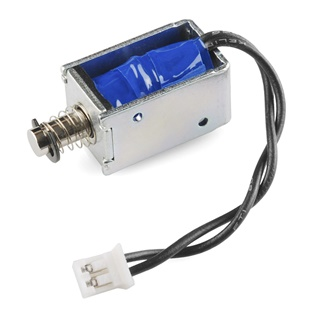Figure 1 - Linear DC actuator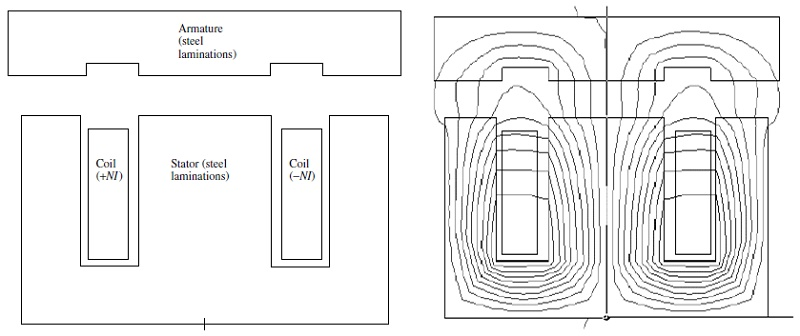Figure 2 - Typical clapper type solenoid actuator: (a) geometry, where steel is made up of thin laminations lying in the plane of the page and stacked in the direction out of the page; (b) computer display of flux lines obtained by finite-element analysis 

## Simulation of a DC actuator using EMWorks

Electro-Magnetic or EM simulation is used to design and study the working of DC linear actuators. It helps to compute the force produced by the work coil, determinate the characteristic of the implemented spring, choose the right materials and optimize the geometric parameters of the actuator.

EMWorks provides both 2D and 3D FEM solutions fully embedded inside SOLIDWORKS. 2D FEM solution is recommended for initial iterative designs because it is quick to perform these types of simulations. A 3D FEM solution is used to confirm the final model parameters before finalizing the design and creating a prototype for field testing.

## Simulation using EMWorks2D inside SOLIDWORKS

### Benchmark 1: Simulation of Clapper Armature Solenoid of Planar Geometry 

In this example, the 2D model is built from basic sketches. The simulated model has a translational invariance along the z axis. The model geometry is shown in Figure 3. The dimensions are w = 10 mm, Al1 = 5 mm, Al2 = 30 mm,
Al3 = 5 mm, Sl1 = 15 mm, Sl2 = 30 mm, Sl3 = 15 mm, and g = 2 mm. The coil is made of copper with 200 turns.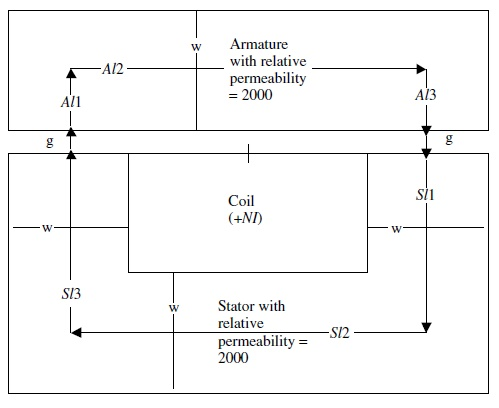Figure 3 - Schematic of the planar clapper armature solenoid

Figure 4 below shows the 2D model meshed in triangles elements. A small mesh size is applied on the air gap. The magnetic flux density is shown in Figure 5 and field lines are shown in Figure 6.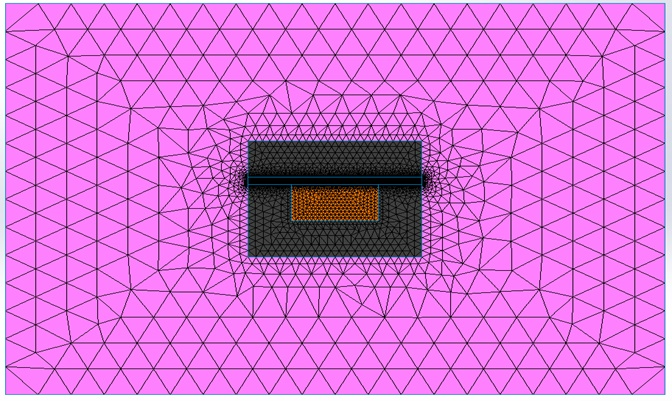Figure 4 - Meshed model for 2D simulation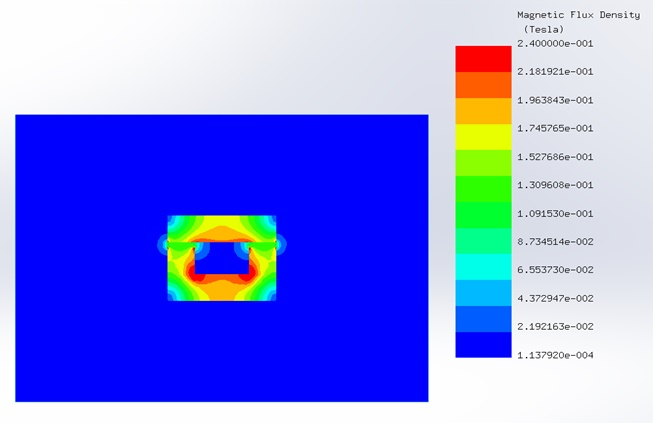Figure 5 - Magnetic flux density distribution in the model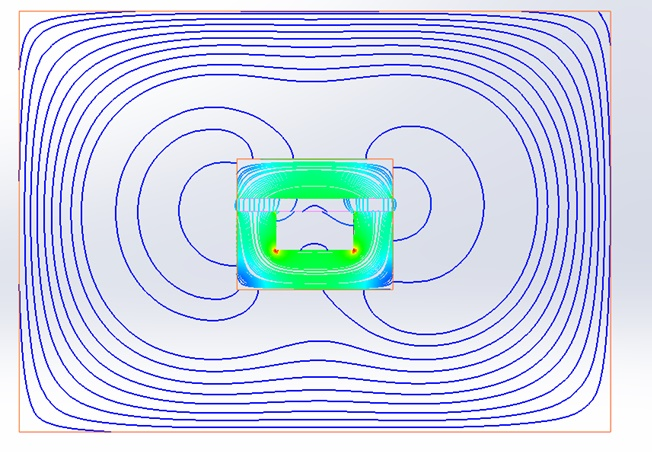Figure 6 - Field lines plot

The force is computed on the moving armature for different currents and air gap lengths. Figure 7 shows the force results computed by EMWorks2D which are in good agreement with reference  results.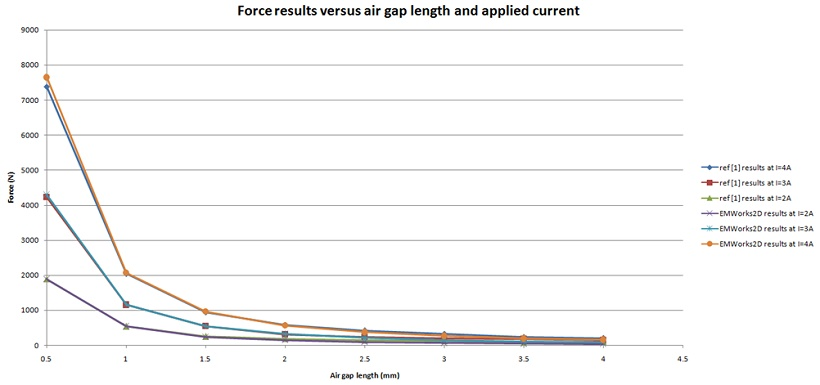Figure 7 - EMWorks2D and reference  results of the magnetic force

### Benchmark 2: 2D Plunger Solenoid Actuator 

This example is treating 3D model of plunger solenoid actuator. The plunger is cylindrical, and the solenoid is axisymmetric. The magnetic force only acts at the end of the plunger. The geometrical parameters are shown in Figure 8.
The number of turns is 400 and the injected current is 4A. The coil is made of copper and the rest of components are made of ferromagnetic material with relative permeability of 2000.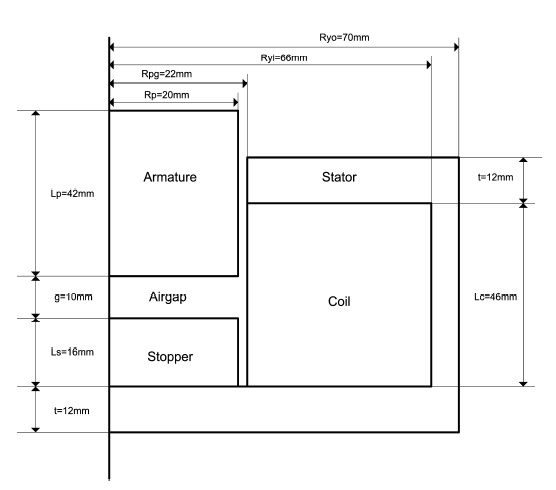Figure 8 - Schematic of the axisymmetric plunger solenoid

A picture of the meshed model is shown in Figure 9.  To adjust the mesh in the air gap, a mesh control with small element size is applied on the air gap.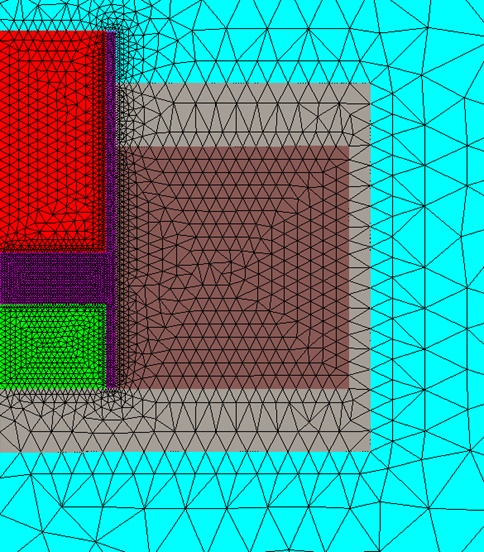Figure 9 - Meshed 2D model

Table 1 shows the computed force for 2 mm air gap given EMWorks2D and reference .

Table 1 - Computed force
 EMWorks2D Ref  Force (N) 19.345 19.34

### Benchmark 3: Force on 3D Clapper Armature Solenoid of Axisymmetric Geometry 

This example represents a revolution symmetry with moving armature. Figure 10 shows the geometry of the axisymmetric actuator. The main dimensions are:
g = 2 mm, wa = 8 mm, R1 = 15 mm, R2 = 25 mm, R3 = 30 mm, Z1 = 8 mm, and Z2 = 23 mm. The coil is made of copper with 2000 turns and excitation DC current of 1A (NI=2000 A.t).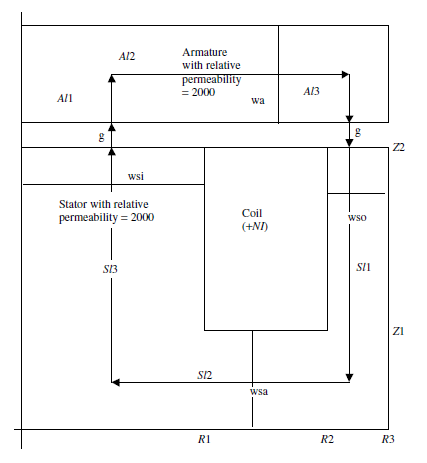Figure 10 - Schematic of the simulated 3D clapper armature with axisymmetric geometry

A section view of the 3D model is shown in Figure 11. The magnetic flux density generated by the DC currents in the actuator is plotted in Figure 12 as vectors.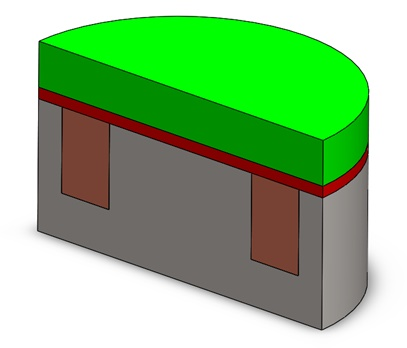Figure 11 - Section view of the 3D model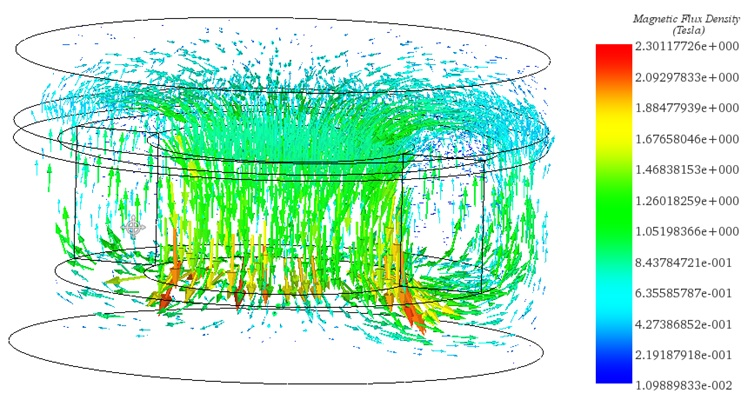Figure 12 - Vector plot of magnetic flux density

Table 2 shows the computed force on the moving armature using EMS for two different relative permeability.
Table 2 - Computed force given by EMS
 Relative permeability 2000 10000 EMS results (N) 283.68 290

Since the previous model represents an axisymmetric geometry, EMWorks2D could be used to solve this problem to minimize the computation time in a preliminary tests. Using the 2D simplification feature in EMWorks2D,
a 3D CAD model is converted easily into 2D surfaces ready for simulation.

Figure 13 shows the direction of magnetic flux density in the 2D model. As a result, the magnetic forces will attract the moving armature to the stator. The magnitude of the attractive force is 279.68 N at 2 mm air gap and 2000 relative permeability.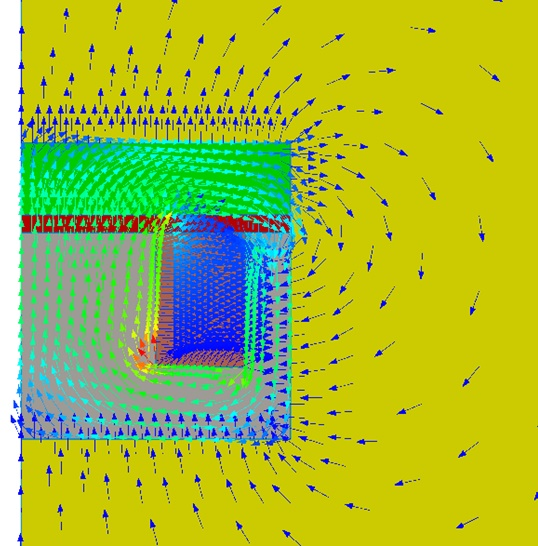Figure 13 - Magnetic flux density in the actuator

Table 3 shows the computed force on the moving armature using EMWorks2D and reference  results. A small error is observed between 2D and 3D results (Table 2) which is due the 2D approximation.

Table 3 - Generated force results for different relative permeability
 Relative permeability EMWorks2D results (N) Reference  results (N) 2000 279.68 279.41 10000 285.42 285.1

## Conclusion

Magnetic force produced by a DC actuator depends on the relative permeability of material, the amperes-turns of coil and the air gap. A 2D simulation by EMWorks2D computes the force and magnetic flux density. The main advantage in a 2D simulation is reduced solving time. Finally, the 3D simulation using EMS, confirms the 2D solution and gives accurate results in more realistic conditions when the 2D assumptions no longer holds true.

#### References

: J. R. Brauer, Magnetic Actuators and Sensors: John Wiley & Sons, 2006.